## 03｜Python科学计算：Pandas 原

Pandas 可以说是基于 NumPy 构建的含有更高级数据结构和分析能力的工具包。在 NumPy 中数据结构是围绕 ndarray 展开的，那么在 Pandas 中的核心数据结构是什么呢？

# 数据结构：Series 和 DataFrame

Series 是个定长的字典序列。说是定长是因为在存储的时候，相当于两个 ndarray，这也是和字典结构最大的不同。因为在字典的结构里，元素的个数是不固定的。
Series 有两个基本属性：index 和 values。在 Series 结构中，index 默认是 0,1,2,……递增的整数序列，当然我们也可以自己来指定索引，比如 index=[‘a’, ‘b’, ‘c’, ‘d’]。

``````import pandas as pd
from pandas import Series, DataFrame
x1 = Series([1,2,3,4])
x2 = Series(data=[1,2,3,4], index=['a', 'b', 'c', 'd'])
print x1
print x2``````

``````0    1
1    2
2    3
3    4
dtype: int64
a    1
b    2
c    3
d    4
dtype: int64``````

``````d = {'a':1, 'b':2, 'c':3, 'd':4}
x3 = Series(d)
print x3 ``````

``````a    1
b    2
c    3
d    4
dtype: int64``````

## DataFrame 类型数据结构类似数据库表。

``````import pandas as pd
from pandas import Series, DataFrame
data = {'Chinese': [66, 95, 93, 90,80],'English': [65, 85, 92, 88, 90],'Math': [30, 98, 96, 77, 90]}
df1= DataFrame(data)
df2 = DataFrame(data, index=['ZhangFei', 'GuanYu', 'ZhaoYun', 'HuangZhong', 'DianWei'], columns=['English', 'Math', 'Chinese'])
print df1
print df2``````

``````            English  Math  Chinese
ZhangFei         65    30       66
GuanYu           85    98       95
ZhaoYun          92    96       93
HuangZhong       88    77       90
DianWei          90    90       80``````

## 数据导入和输出

Pandas 允许直接从 xlsx，csv 等文件中导入数据，也可以输出到 xlsx, csv 等文件，非常方便。

``````import pandas as pd
from pandas import Series, DataFrame
score.to_excel('data1.xlsx')
print score``````

## 数据清洗

``````data = {'Chinese': [66, 95, 93, 90,80],'English': [65, 85, 92, 88, 90],'Math': [30, 98, 96, 77, 90]}
df2 = DataFrame(data, index=['ZhangFei', 'GuanYu', 'ZhaoYun', 'HuangZhong', 'DianWei'], columns=['English', 'Math', 'Chinese'])``````

### 1. 删除 DataFrame 中的不必要的列或行

Pandas 提供了一个便捷的方法 drop() 函数来删除我们不想要的列或行。比如我们想把“语文”这列删掉。

``df2 = df2.drop(columns=['Chinese'])``

``df2 = df2.drop(index=['ZhangFei'])``

### 2. 重命名列名 columns，让列表名更容易识别

``df2.rename(columns={'Chinese': 'YuWen', 'English': 'Yingyu'}, inplace = True)``

### 3. 去重复的值

``df = df.drop_duplicates() #去除重复行``

### 4. 格式问题

#### 更改数据格式

``````df2['Chinese'].astype('str')
df2['Chinese'].astype(np.int64) ``````

#### 数据间的空格

``````#删除左右两边空格
df2['Chinese']=df2['Chinese'].map(str.strip)
#删除左边空格
df2['Chinese']=df2['Chinese'].map(str.lstrip)
#删除右边空格
df2['Chinese']=df2['Chinese'].map(str.rstrip)``````

``df2['Chinese']=df2['Chinese'].str.strip('\$')``

#### 大小写转换

``````#全部大写
df2.columns = df2.columns.str.upper()
#全部小写
df2.columns = df2.columns.str.lower()
#首字母大写
df2.columns = df2.columns.str.title()``````

#### 查找空值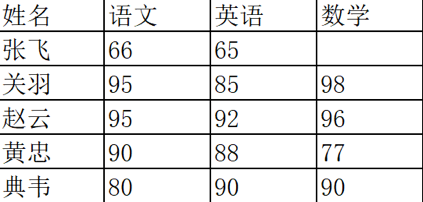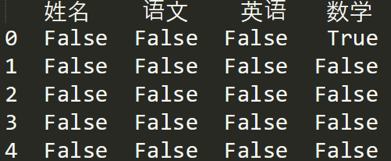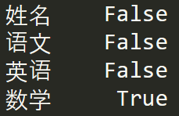#### 使用 apply 函数对数据进行清洗

apply 函数是 Pandas 中自由度非常高的函数，使用频率也非常高。

``df['name'] = df['name'].apply(str.upper)``

``````def double_df(x):
return 2*x
df1[u'语文'] = df1[u'语文'].apply(double_df)``````

``````def plus(df,n,m):
df['new1'] = (df[u'语文']+df[u'英语']) * m
df['new2'] = (df[u'语文']+df[u'英语']) * n
return df
df1 = df1.apply(plus,axis=1,args=(2,3,))``````

### 数据统计

Pandas 和 NumPy 一样，都有常用的统计函数，如果遇到空值 NaN，会自动排除。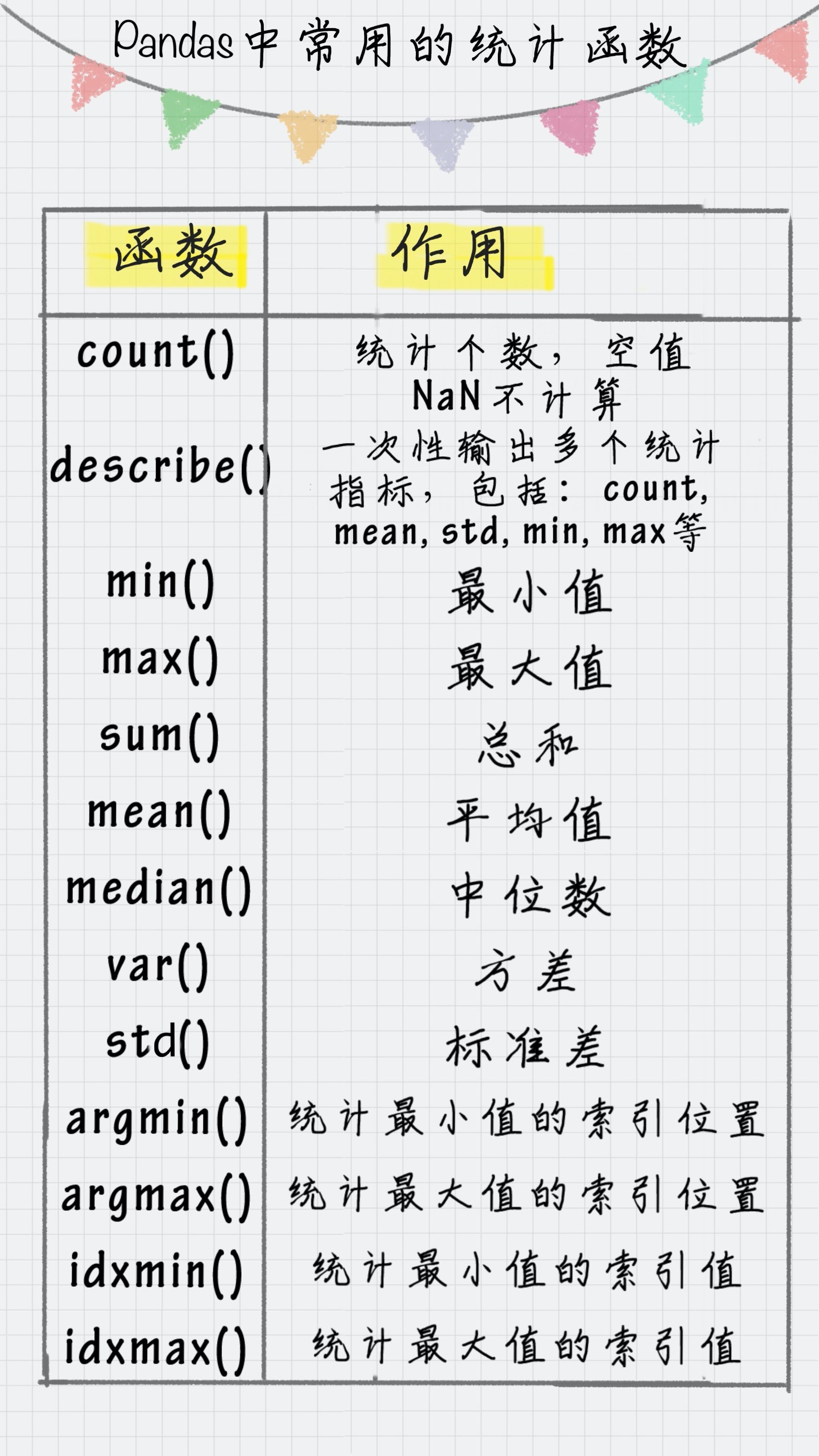``````df1 = DataFrame({'name':['ZhangFei', 'GuanYu', 'a', 'b', 'c'], 'data1':range(5)})
print df1.describe()``````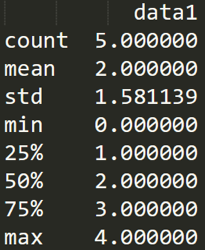### 数据表合并

``````df1 = DataFrame({'name':['ZhangFei', 'GuanYu', 'a', 'b', 'c'], 'data1':range(5)})
df2 = DataFrame({'name':['ZhangFei', 'GuanYu', 'A', 'B', 'C'], 'data2':range(5)})``````

#### 1. 基于指定列进行连接

``df3 = pd.merge(df1, df2, on='name')``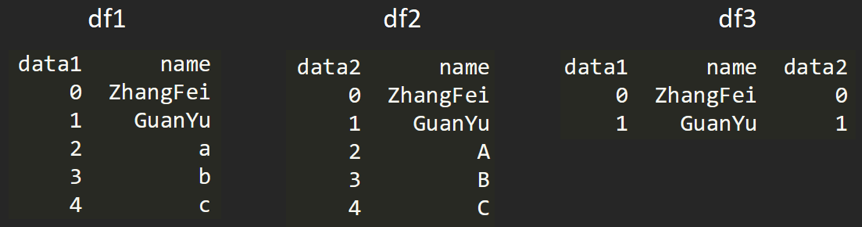#### 2. inner 内连接

inner 内链接是 merge 合并的默认情况，inner 内连接其实也就是键的交集，在这里 df1, df2 相同的键是 name，所以是基于 name 字段做的连接：

``df3 = pd.merge(df1, df2, how='inner')``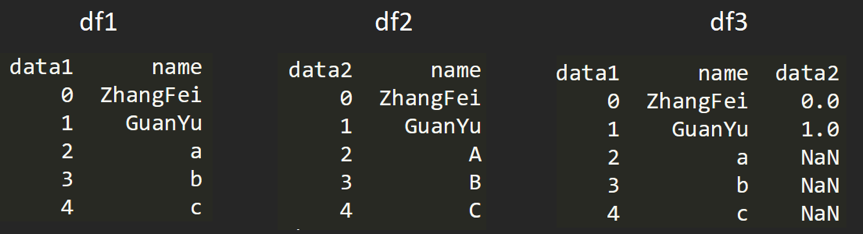#### 4. right 右连接

``df3 = pd.merge(df1, df2, how='right')``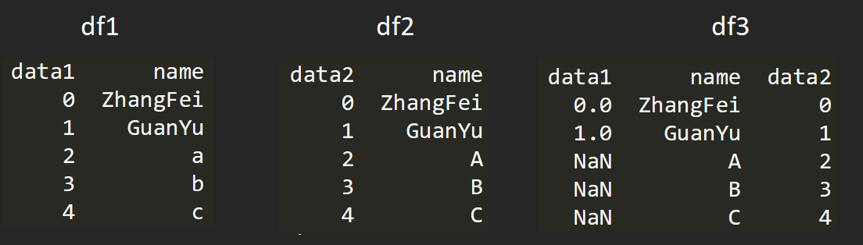#### 5. outer 外连接

``df3 = pd.merge(df1, df2, how='outer')``

### 如何用 SQL 方式打开 Pandas

Pandas 的 DataFrame 数据类型可以让我们像处理数据表一样进行操作，比如数据表的增删改查，都可以用 Pandas 工具来完成。不过也会有很多人记不住这些 Pandas 的命令，相比之下还是用 SQL 语句更熟练，用 SQL 对数据表进行操作是最方便的，它的语句描述形式更接近我们的自然语言。

pandasql 中的主要函数是 sqldf，它接收两个参数：一个 SQL 查询语句，还有一组环境变量 globals() 或 locals()。这样我们就可以在 Python 里，直接用 SQL 语句中对 DataFrame 进行操作，举个例子：

``````import pandas as pd
from pandas import DataFrame
df1 = DataFrame({'name':['ZhangFei', 'GuanYu', 'a', 'b', 'c'], 'data1':range(5)})
pysqldf = lambda sql: sqldf(sql, globals())
sql = "select * from df1 where name ='ZhangFei'"
print pysqldf(sql)``````

``````   data1      name
0      0  ZhangFei``````

``lambda argument_list: expression``

``pysqldf = lambda sql: sqldf(sql, globals())``

# 总结

Pandas 包与 NumPy 工具库配合使用可以发挥巨大的威力，正是有了 Pandas 工具，Python 做数据挖掘才具有优势。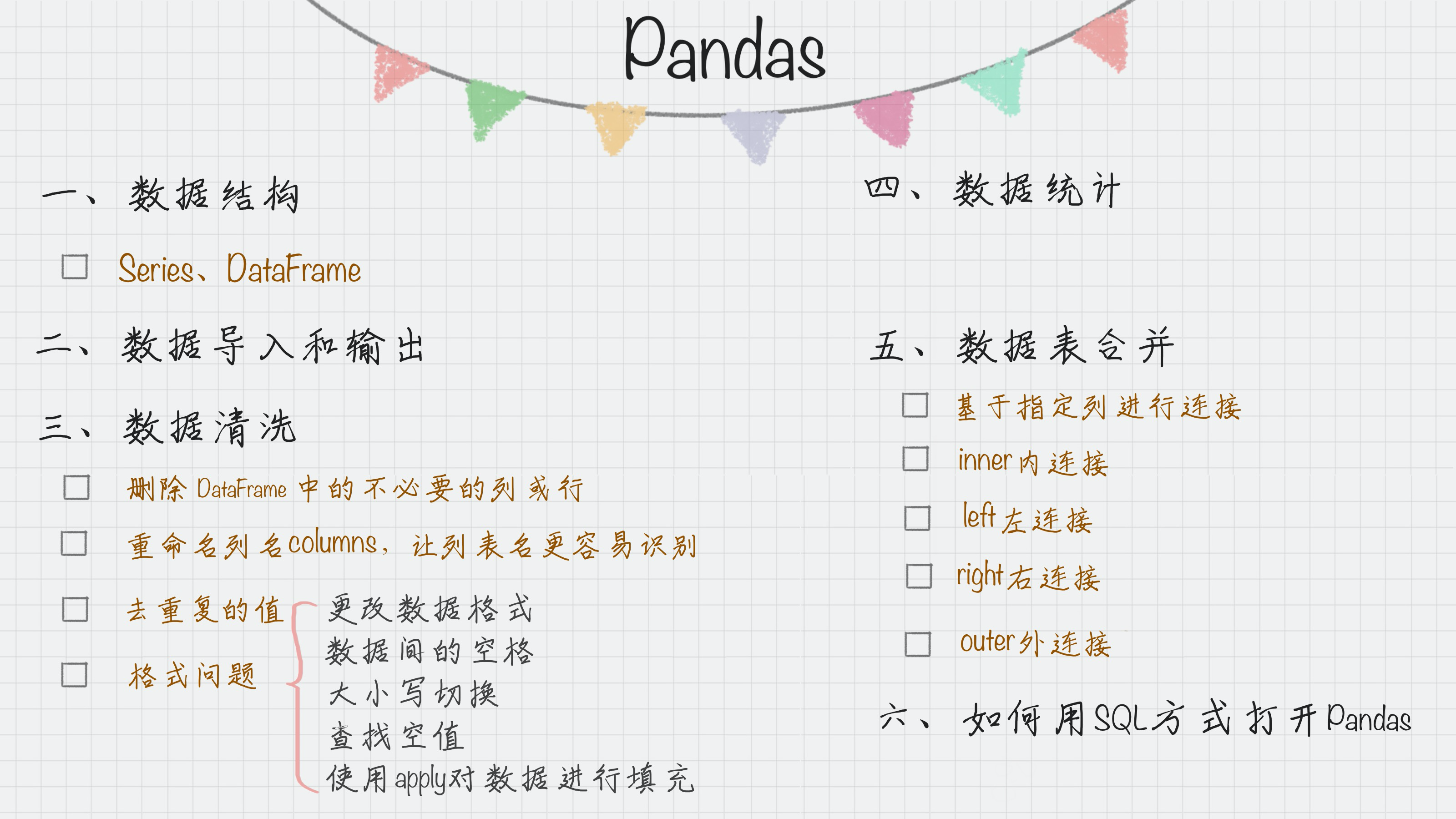### 评论(0)

IT界的小小小学生
03/31
0
0

IT程序员
2019/05/13
0
0

TIOBE 最新发布的 9 月编程语言排行榜中，Python 凭 4.67% 的增速以 0.26% 的优势力压 C++，逆袭成功进入 Top 3。 TIOBE 9 月编程语言 Top 5 而近一年势头不灭的 Python 在数据分析领域，是专...

CSDN资讯
2018/09/09
0
0

2019/09/30
0
0

Python是目前学习数据分析课程中，非常重要的一块，也是目前非常受行业欢迎的。因此，学习非常重要，下面是Python学习的一些重要步骤和知识！ 步骤1：熟悉Python 对数据科学，Python和R都是不...

weixin_41852491
2018/05/28
0
0

osc_6n4iy0i5
21分钟前
45
0
element-ui 响应式布局-栅格布局

osc_7ehwx8hw
22分钟前
33
0

osc_z3ivsxnp
23分钟前
35
0
【Q&A干货分享】敲重点，企业必须关注的等保灵魂20问

osc_1ajf1srl
25分钟前
31
0

osc_cgllnrkd
26分钟前
70
0